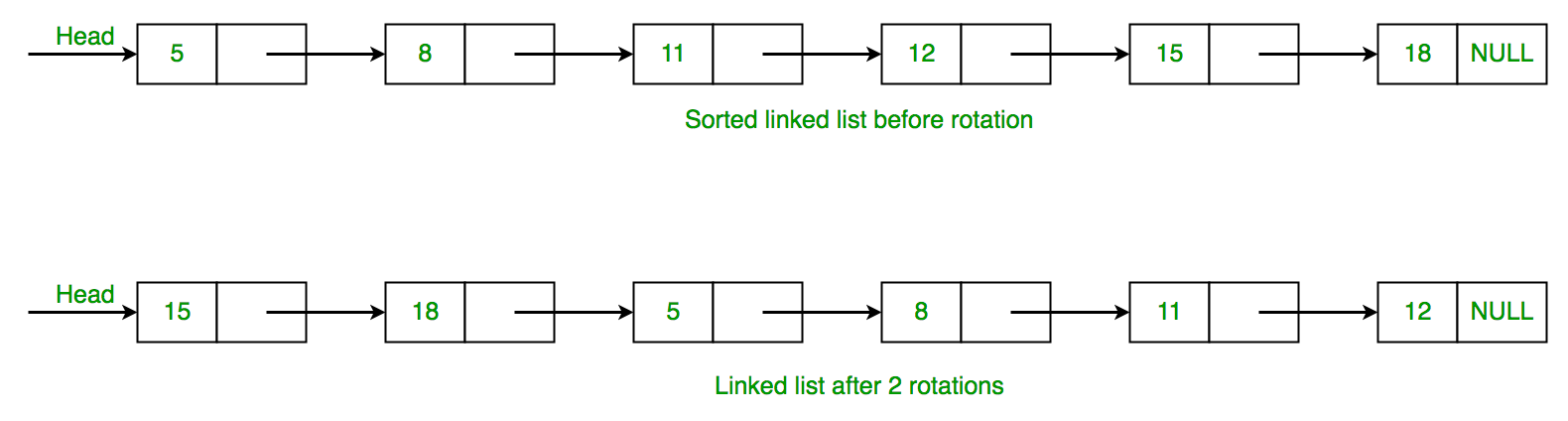GeeksforGeeks App
Open AppBrowser
Continue

# Java Program to Count rotations in sorted and rotated linked list

Given a linked list of n nodes which is first sorted, then rotated by k elements. Find the value of k.The idea is to traverse singly linked list to check condition whether current node value is greater than value of next node. If the given condition is true, then break the loop. Otherwise increase the counter variable and increase the node by node->next. Below is the implementation of this approach.

## Java

 `// Program for count number of rotations in``// sorted linked list.``import` `java.util.*;` `class` `GFG``{``  ` `/* Linked list node */``static` `class` `Node {``    ``int` `data;``    ``Node next;``};` `// Function that count number of``// rotation in singly linked list.``static` `int` `countRotation(Node head)``{``  ` `    ``// declare count variable and assign it 1.``    ``int` `count = ``0``;` `    ``// declare a min variable and assign to``    ``// data of head node.``    ``int` `min = head.data;` `    ``// check that while head not equal to null.``    ``while` `(head != ``null``) {` `        ``// if min value is greater then head.data``        ``// then it breaks the while loop and``        ``// return the value of count.``        ``if` `(min > head.data)``            ``break``;` `        ``count++;` `        ``// head assign the next value of head.``        ``head = head.next;``    ``}``    ``return` `count;``}` `// Function to push element in linked list.``static` `Node push(Node head, ``int` `data)``{``    ``// Allocate dynamic memory for newNode.``    ``Node newNode = ``new` `Node();` `    ``// Assign the data into newNode.``    ``newNode.data = data;` `    ``// newNode.next assign the address of``    ``// head node.``    ``newNode.next = (head);` `    ``// newNode become the headNode.``    ``(head) = newNode;``    ``return` `head;``}` `// Display linked list.``static` `void` `printList(Node node)``{``    ``while` `(node != ``null``) {``        ``System.out.printf(``"%d "``, node.data);``        ``node = node.next;``    ``}``}` `// Driver functions``public` `static` `void` `main(String[] args)``{``  ` `    ``// Create a node and initialize with null``    ``Node head = ``null``;` `    ``// push() insert node in linked list.``    ``// 15.18.5.8.11.12``    ``head = push(head, ``12``);``    ``head = push(head, ``11``);``    ``head = push(head, ``8``);``    ``head = push(head, ``5``);``    ``head = push(head, ``18``);``    ``head = push(head, ``15``);` `    ``printList(head);``    ``System.out.println();``    ``System.out.print(``"Linked list rotated elements: "``);` `    ``// Function call countRotation()``    ``System.out.print(countRotation(head) +"``");` `}``}` `// This code contributed by gauravrajput1`

Output

```15 18 5 8 11 12